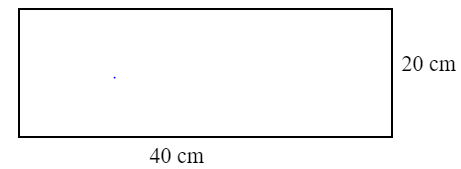QUESTION

# Find the perimeter of the following figure :Hint: In this question , use $2(l + b)$ i.e. the perimeter of a rectangle to find the perimeter of the given figure.
Length of the rectangle $(l) = 40cm$
Breadth of the rectangle $(b) = 20cm$
We know that perimeter of rectangle $= 2(l + b)$
Hence , substituting the value of $l$ and $b$ in the formula we get,
$= 2(40 + 20) \\ = 2(60) \\ = 120cm \\$
$\therefore$ The perimeter of the figure is $120cm$.